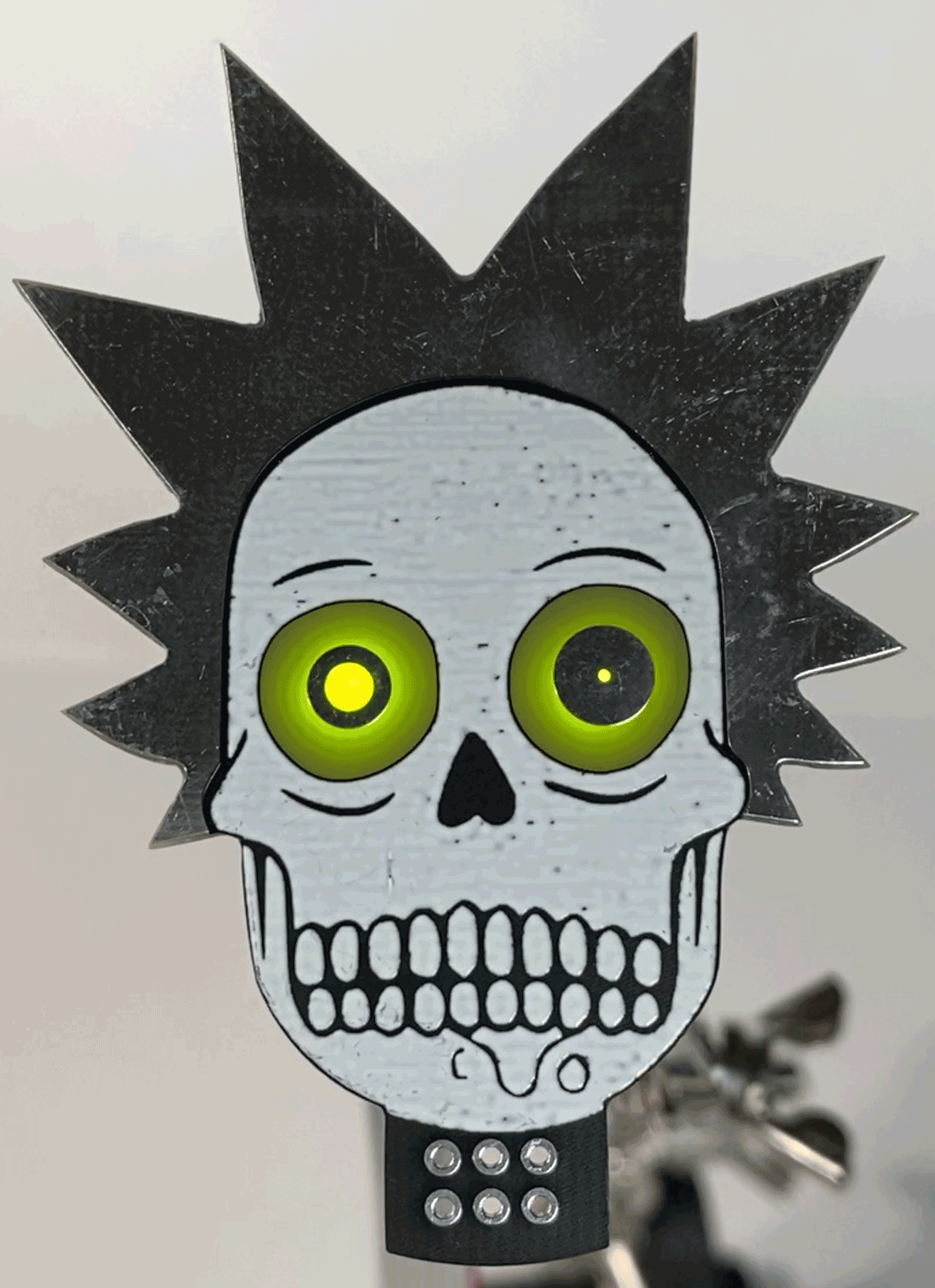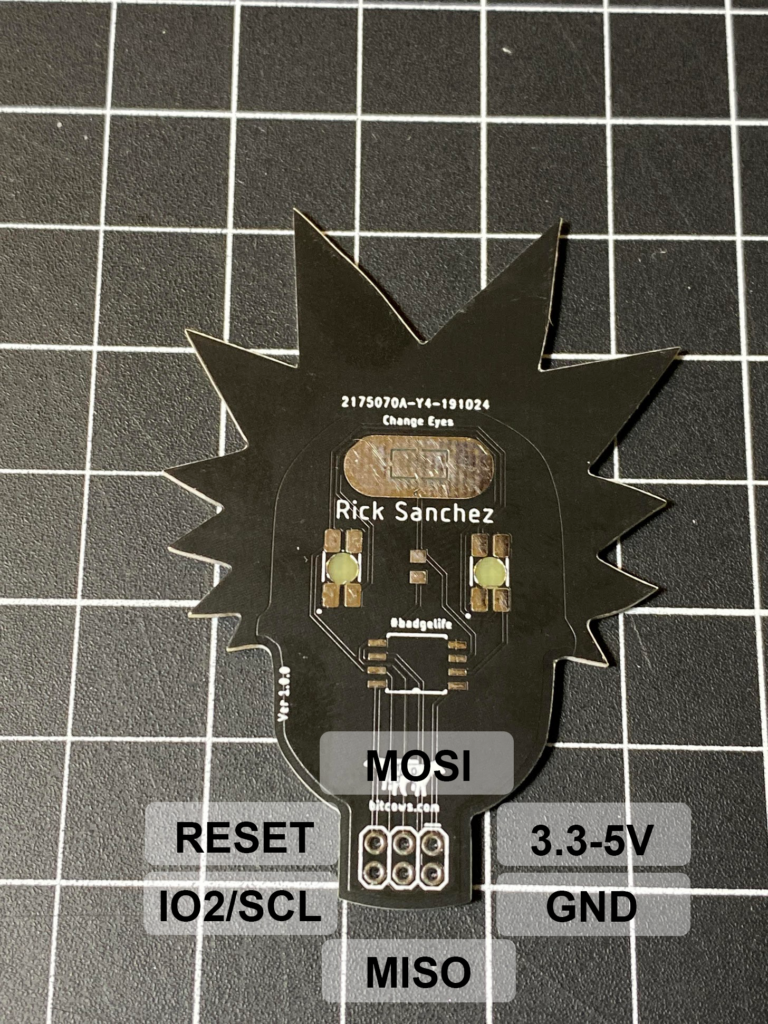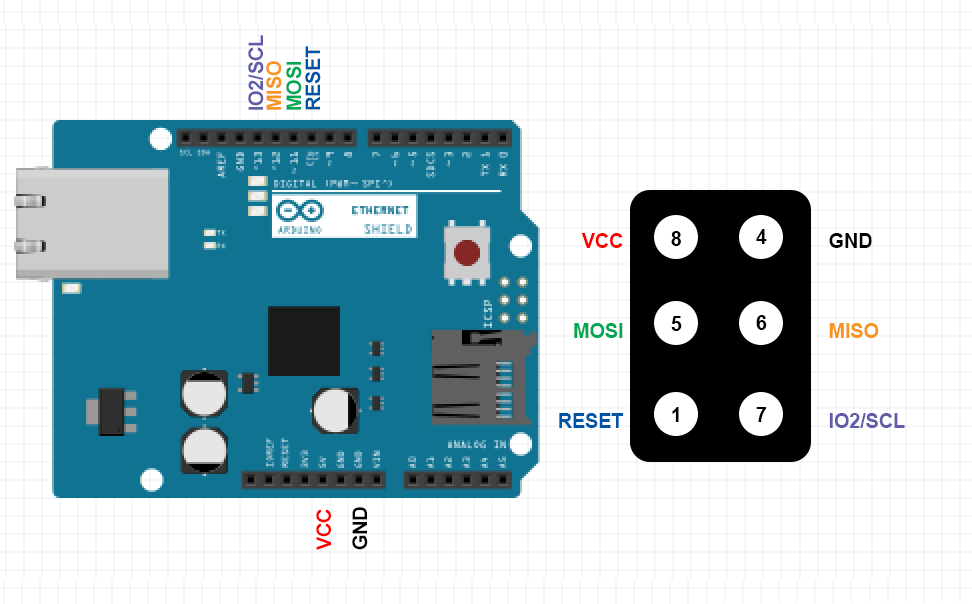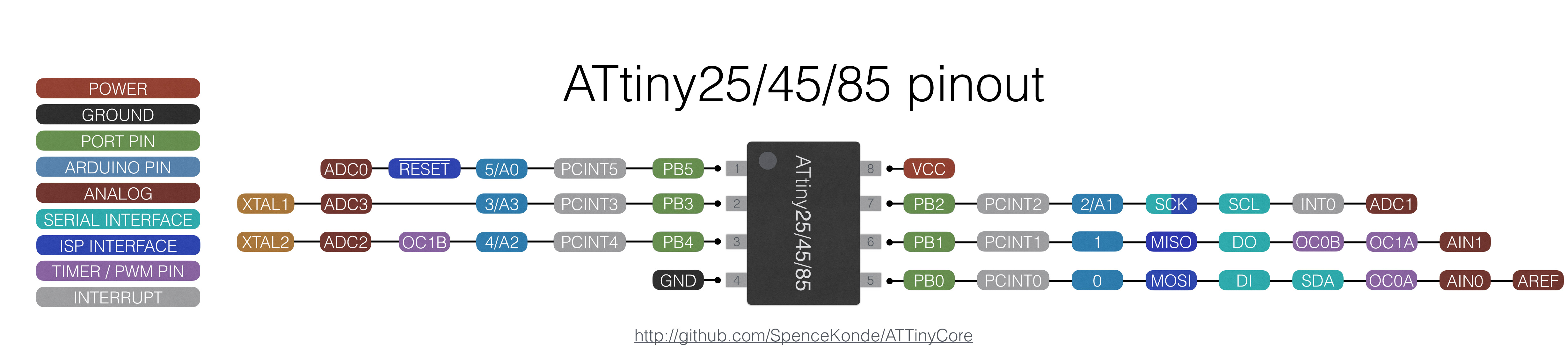### SAO Instructions# Pinout for version 1.0.2# Arduino

You can use the Arduino to program the board. However in order to program this directly you will need a 8mhz crystal on pin 2 and 3 of the ATtiny85.You might want to look at these articals for more information on prgraming an ATTiny84

Program a ATtiny85 SOIC 8 using an Arduino

# Arduino Code

```/*

Darren Mason
bitcows.com
10/29/2019

*/
unsigned long csSum;
int looptype = 1;
int orange = 3; //IO1 pin 7
int green = 2; //IO3 pin 2

int state = HIGH;
int r;
int p = LOW;
long time = 0;
long debounce = 200;

void setup()
{
pinMode(orange, OUTPUT);
pinMode(green, OUTPUT);
}

void loop()
{

//8mhz
if (looptype == 0) { //blink orange and green
digitalWrite(orange, HIGH);
delay(20);
digitalWrite(orange, LOW);
delay(20);
digitalWrite(green, HIGH);
delay(20);
digitalWrite(green, LOW);

} else if (looptype == 1) { //hyperflash both
digitalWrite(orange, HIGH);
delay(5);
digitalWrite(orange, LOW);
delay(0);
digitalWrite(green, HIGH);
delay(5);
digitalWrite(green, LOW);
} else if (looptype == 2) { //blink Green
digitalWrite(green, HIGH);
delay(10);
digitalWrite(green, LOW);
delay(10);
} else if (looptype == 3) { //slow flash both
digitalWrite(orange, HIGH);
delay(10);
digitalWrite(orange, LOW);
delay(10);
}else if (looptype == 4){
digitalWrite(green, HIGH);
}else {
digitalWrite(orange, HIGH);
}

if (r == HIGH && p == LOW && millis() - time > debounce) {
if (state == HIGH && looptype <= 5)
looptype = looptype + 1;
else
looptype = 0;

time = millis();
delay(200);
}

p = r;
}

```